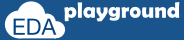# Static Delay

```a ##2 b;
```

If signal "a" is high on any given positive edge of the clock, the signal "b" should be high 2 clock cycles after that.

## Variable Delay

```int v_delay;
v_delay = 4;```
`a ##v_delay b;`

Using ##v_delay leads to compilation error because it is Illegal to use a variable in an assertion.

```module asertion_variable_delay;
bit clk,a,b;
int cfg_delay;

always #5 clk = ~clk; //clock generation

//generating 'a'
initial begin
cfg_delay = 4;
a=1; b = 0;
#15 a=0; b = 1;
#10 a=1;
#10 a=0; b = 1;
#10 a=1; b = 0;
#10;
\$finish;
end

//calling assert property
a_1: assert property(@(posedge clk) a ##cfg_delay b);

endmodule  ```

Simulator Output

Error-[SVA-INCE] Illegal use of non-constant expression
testbench.sv, 23
asertion_variable_delay, "cfg_delay"
The use of a non-constant expression is not allowed in properties, sequences
and assertions for cases such as delay and repetition ranges.
Please replace the offending expression by an elaboration-time constant.

1 error

Execute the above code on### Below is one of the ways to implement the variable delay

```module asertion_variable_delay;
bit clk,a,b;
int cfg_delay;

always #5 clk = ~clk; //clock generation

//generating 'a'
initial begin
cfg_delay  = 4;
a=1; b = 0;
#15 a=0; b = 1;
#10 a=1;
#10 a=0; b = 1;
#10 a=1; b = 0;
#10;
\$finish;
end

//delay sequence
sequence delay_seq(v_delay);
int delay;
(1,delay=v_delay) ##0 first_match((1,delay=delay-1) [*0:\$] ##0 delay <=0);
endsequence

//calling assert property
a_1: assert property(@(posedge clk) a |-> delay_seq(cfg_delay) |-> b);

endmodule
```

```a |-> delay_seq(cfg_delay) |-> b;

sequence delay_seq(v_delay);
int delay;
(1,delay=v_delay) ##0 first_match((1,delay=delay-1) [*0:\$] ##0 delay <=0);
endsequence
```

Instead of ##v_delay, sequence 'delay_seq' is used for variable delay.
delay_seq works like while loop, variable value will be decremented on each clk cycle and checks for the value of 'delay' equals to '0'. The sequence will get ended once the value of 'delay' equals '0'.
• (1,delay=v_delay)                                    -> Copy variable value to local variable.
• (1,delay=delay-1) [*0:\$] ##0 delay <=0 -> Decrements the value of local variable and checks for value of 'delay' equals to '0'.
• first_match((1,delay=delay-1) [*0:\$] ##0 delay <=0) -> waits for value of 'delay' equals to '0'

Execute the above code on